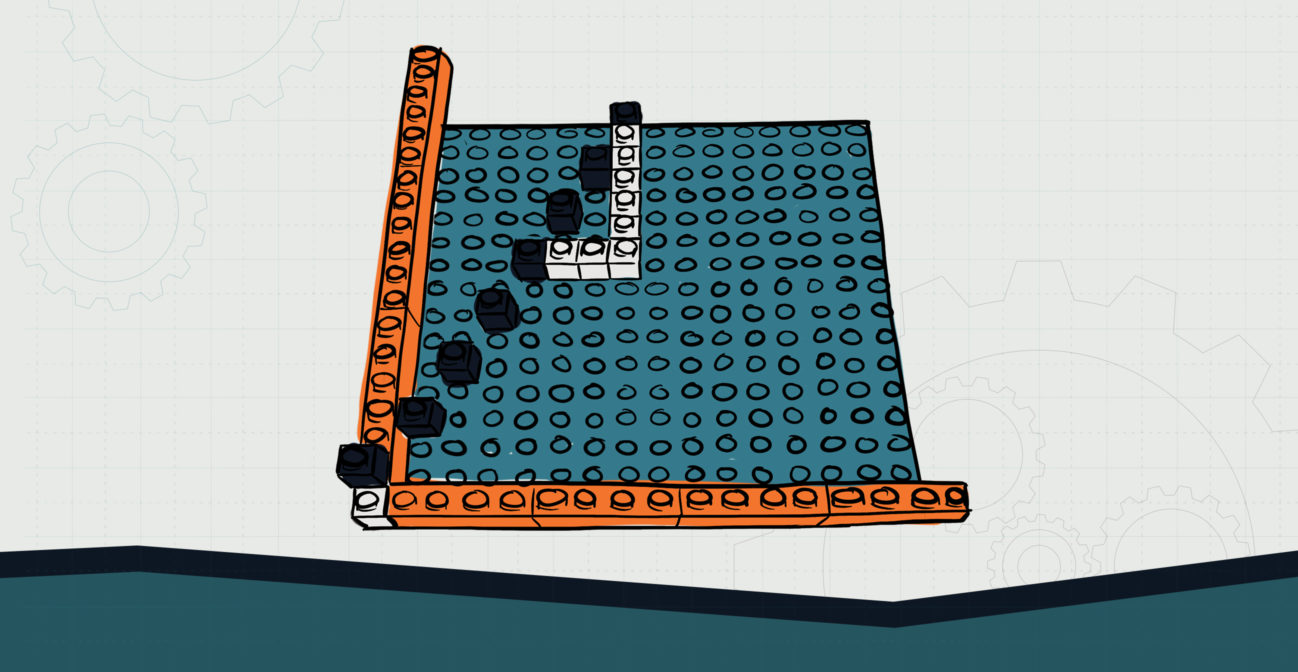# The Plots Thicken

## Model coordinate planes and plot using bricks.Objective:
Child will be able to practice plotting points on a coordinate plane.

Essential Question:
How might we plot points in a coordinate plane?

Special Materials:
None

Bricks Required:
1x bricks of various sizes; two brick plates

Project Structure

Engage/Explain:

1. Ask child to create a horizontal number line extending in both – and + directions. Ask that the zero be a different color brick.
1. Have child practice plotting numbers along this number line (a skill they should already have).
2. Monitor child and check for misconceptions.
2. Have child create a vertical number line using the same zero point and extending in both – and + directions.
1. Child will have created an x-y coordinate system.
3. Have child practice plotting on the horizontal and vertical axes separately to make sure they are able to identify each axis. You may choose to refer to these as vertical/horizontal, up-down/left-right, or as x and y.
4. Once child gets the hang of things, provide them coordinates in pairs (x,y).
1. As child plots each point, circulate and check for and correct misconceptions.

Explore:

1. Have child clear their graph of any previous points.
2. Provide child a set of coordinates:
1. (2,2) (-2,2) (2,-2) (-2,-2)
3. Ask child to plot these points and reflect upon them.
1. What is different about them?
2. What happens to the point when a negative is added?